This book is archived and will be removed July 6, 2022. Please use the updated version.

Standard Costs and Variances

# 47 Compute and Evaluate Labor Variances

In addition to evaluating materials usage, companies must assess how efficiently and effectively they are using labor in the production of their products. Direct labor is a cost associated with workers working directly in the production process. The company must look at both the quantity of hours used and the rate of the labor and compare outcomes to standard costs. Determining efficiency and effectiveness of labor leads to individual labor variances. A company can compute these labor variances and make informed decisions about labor operations based on these differences.

### Fundamentals of Direct Labor Variances

The direct labor variance measures how efficiently the company uses labor as well as how effective it is at pricing labor. There are two components to a labor variance, the direct labor rate variance and the direct labor time variance.

#### Direct Labor Rate Variance

The direct labor rate variance compares the actual rate per hour of direct labor to the standard rate per hour of labor for the hours worked. The direct labor rate variance is calculated using this formula: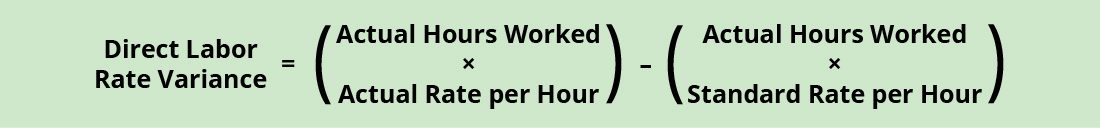Factoring out the actual hours worked from both components of the formula, it can be rewritten asWith either of these formulas, the actual rate per hour refers to the actual rate of pay for workers to create one unit of product. The standard rate per hour is the expected rate of pay for workers to create one unit of product. The actual hours worked are the actual number of hours worked to create one unit of product. If there is no difference between the standard rate and the actual rate, the outcome will be zero, and no variance exists.

If the actual rate of pay per hour is less than the standard rate of pay per hour, the variance will be a favorable variance. A favorable outcome means you paid workers less than anticipated. If, however, the actual rate of pay per hour is greater than the standard rate of pay per hour, the variance will be unfavorable. An unfavorable outcome means you paid workers more than anticipated.

The actual rate can differ from the standard or expected rate because of supply and demand of the workers, increased labor costs due to economic changes or union contracts, or the ability to hire employees at a different skill level. Once the manufacturer makes the products, the labor costs will follow the goods through production, so the company should evaluate how the difference between what it expected to happen and what actually happened will affect all the goods produced using these particular labor rates.

Let us again consider Connie’s Candy Company with respect to labor. Connie’s Candy establishes a standard rate per hour for labor of ?8.00. Each box of candy is expected to require 0.10 hours of labor (6 minutes). Connie’s Candy found that the actual rate of pay per hour for labor was ?7.50. They still actually required 0.10 hours of labor to make each box. The direct labor rate variance computes as:

$$\text{Direct Labor Rate Variance}=\left(?7.50–?8.00\right)\phantom{\rule{0.2em}{0ex}}\text{×}\phantom{\rule{0.2em}{0ex}}0.10\phantom{\rule{0.2em}{0ex}}\text{hours}=–?0.05\phantom{\rule{0.2em}{0ex}}\text{or}\phantom{\rule{0.2em}{0ex}}?0.05\phantom{\rule{0.2em}{0ex}}\left(\text{Favorable}\right)$$

In this case, the actual rate per hour is ?7.50, the standard rate per hour is ?8.00, and the actual hour worked is 0.10 hours per box. This computes as a favorable outcome. This is a favorable outcome because the actual rate of pay was less than the standard rate of pay. As a result of this favorable outcome information, the company may consider continuing operations as they exist, or could change future budget projections to reflect higher profit margins, among other things.

Let us take the same example except now the actual rate of pay per hour is ?9.50. The direct labor rate variance computes as:

$$\text{Direct Labor Rate Variance}=\left(?9.50–?8.00\right)\phantom{\rule{0.2em}{0ex}}\text{×}\phantom{\rule{0.2em}{0ex}}0.10\phantom{\rule{0.2em}{0ex}}\text{hours}=?0.15\phantom{\rule{0.2em}{0ex}}\text{or}\phantom{\rule{0.2em}{0ex}}?0.15\phantom{\rule{0.2em}{0ex}}\left(\text{Unfavorable}\right)$$

In this case, the actual rate per hour is ?9.50, the standard rate per hour is ?8.00, and the actual hours worked per box are 0.10 hours. This computes as an unfavorable outcome. This is an unfavorable outcome because the actual rate per hour was more than the standard rate per hour. As a result of this unfavorable outcome information, the company may consider using cheaper labor, changing the production process to be more efficient, or increasing prices to cover labor costs.

Another element this company and others must consider is a direct labor time variance.

#### Direct Labor Time Variance

The direct labor time variance compares the actual labor hours used to the standard labor hours that were expected to be used to make the actual units produced. The variance is calculated using this formula:Factoring out the standard rate per hour from both components of the formula, it can be rewritten as:With either of these formulas, the actual hours worked refers to the actual number of hours used at the actual production output. The standard rate per hour is the expected hourly rate paid to workers. The standard hours are the expected number of hours used at the actual production output. If there is no difference between the actual hours worked and the standard hours, the outcome will be zero, and no variance exists.

If the actual hours worked are less than the standard hours at the actual production output level, the variance will be a favorable variance. A favorable outcome means you used fewer hours than anticipated to make the actual number of production units. If, however, the actual hours worked are greater than the standard hours at the actual production output level, the variance will be unfavorable. An unfavorable outcome means you used more hours than anticipated to make the actual number of production units.

The actual hours used can differ from the standard hours because of improved efficiencies in production, carelessness or inefficiencies in production, or poor estimation when creating the standard usage.

Consider the previous example with Connie’s Candy Company. Connie’s Candy establishes a standard rate per hour for labor of ?8.00. Each box of candy is expected to require 0.10 hours of labor (6 minutes). Connie’s Candy found that the actual hours worked per box were 0.05 hours (3 minutes). The actual rate per hour for labor remained at ?8.00 to make each box. The direct labor time variance computes as:

$$\text{Direct Labor Time Variance}=\left(0.05–0.10\right)\phantom{\rule{0.2em}{0ex}}\text{×}\phantom{\rule{0.2em}{0ex}}?8.00\phantom{\rule{0.2em}{0ex}}\text{per hour}=–?0.40\phantom{\rule{0.2em}{0ex}}\text{or}\phantom{\rule{0.2em}{0ex}}?0.40\phantom{\rule{0.2em}{0ex}}\left(\text{Favorable}\right)$$

In this case, the actual hours worked are 0.05 per box, the standard hours are 0.10 per box, and the standard rate per hour is ?8.00. This computes as a favorable outcome. This is a favorable outcome because the actual hours worked were less than the standard hours expected. As a result of this favorable outcome information, the company may consider continuing operations as they exist, or could change future budget projections to reflect higher profit margins, among other things.

Let us take the same example except now the actual hours worked are 0.20 hours per box. The direct labor time variance computes as:

$$\text{Direct Labor Time Variance}=\left(?0.20–?0.10\right)\phantom{\rule{0.2em}{0ex}}\text{×}\phantom{\rule{0.2em}{0ex}}?8.00\phantom{\rule{0.2em}{0ex}}\text{per hour}=?0.80\phantom{\rule{0.2em}{0ex}}\text{or}\phantom{\rule{0.2em}{0ex}}?0.80\phantom{\rule{0.2em}{0ex}}\left(\text{Unfavorable}\right)$$

In this case, the actual hours worked per box are 0.20, the standard hours per box are 0.10, and the standard rate per hour is ?8.00. This computes as an unfavorable outcome. This is an unfavorable outcome because the actual hours worked were more than the standard hours expected per box. As a result of this unfavorable outcome information, the company may consider retraining its workers, changing the production process to be more efficient, or increasing prices to cover labor costs.

The combination of the two variances can produce one overall total direct labor cost variance.

Package Deliveries

UPS drivers are evaluated on how many miles they drive and how quickly they deliver packages. The drivers are given the route and time they are expected to take, so they are expected to complete their route in a timely and efficient manner. They also work until all packages are delivered. A GPS tracking system tracks the trucks throughout the day. The system keeps track of how much they back up and if they take any left turns because right turns are much more time efficient.1 Tracking drivers like this does not leave them very much time to deal with customers. Customer service is a major part of the driver’s job. Can the driver service the customer and drive the route in the time and distance allotted? Which is more important: customer service or driving the route in a timely and efficient manner?

#### Total Direct Labor Variance

When a company makes a product and compares the actual labor cost to the standard labor cost, the result is the total direct labor variance.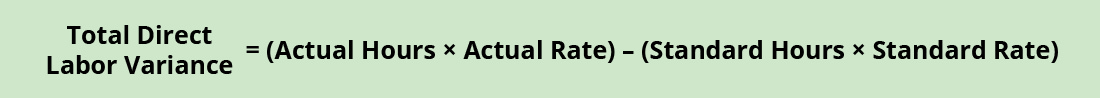If the outcome is unfavorable, the actual costs related to labor were more than the expected (standard) costs. If the outcome is favorable, the actual costs related to labor are less than the expected (standard) costs.

The total direct labor variance is also found by combining the direct labor rate variance and the direct labor time variance. By showing the total direct labor variance as the sum of the two components, management can better analyze the two variances and enhance decision-making.

(Figure) shows the connection between the direct labor rate variance and direct labor time variance to total direct labor variance.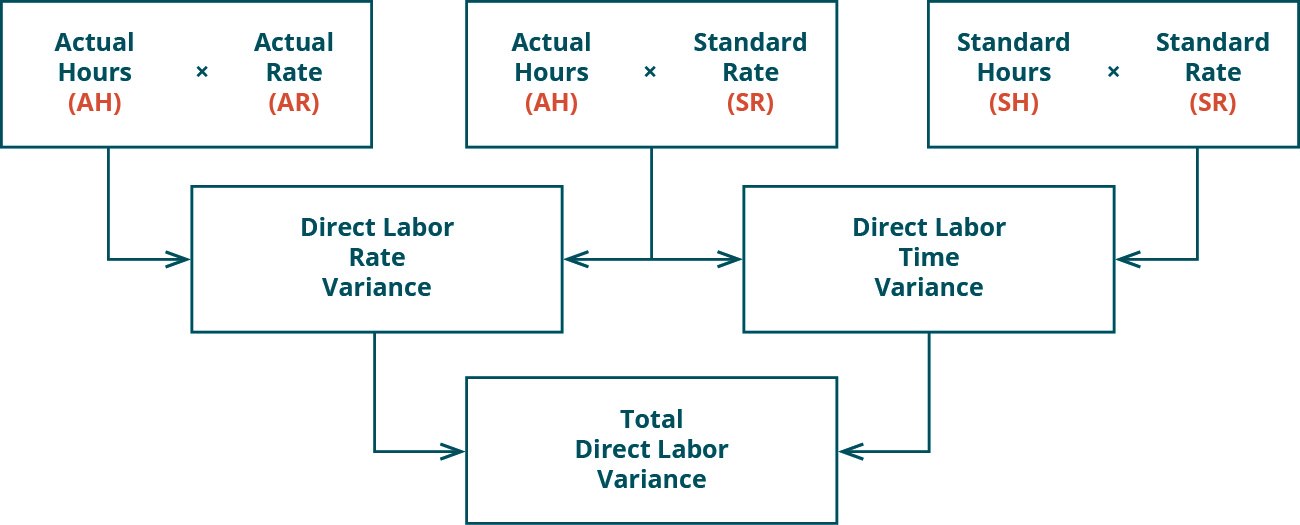For example, Connie’s Candy Company expects to pay a rate of ?8.00 per hour for labor but actually pays ?9.50 per hour. The company expected to use 0.10 hours of labor per box but actually used 0.20 hours per box. The total direct labor variance is computed as:

$$\text{Total Direct Labor Time Variance}=\left(0.20\phantom{\rule{0.2em}{0ex}}\text{hours}\phantom{\rule{0.2em}{0ex}}×\phantom{\rule{0.2em}{0ex}}?9.50\right)–\left(0.10\phantom{\rule{0.2em}{0ex}}\text{hours}\phantom{\rule{0.2em}{0ex}}\text{×}\phantom{\rule{0.2em}{0ex}}?8.00\right)=?1.90–?0.80=?1.10\phantom{\rule{0.2em}{0ex}}\left(\text{Unfavorable}\right)$$

In this case, two elements are contributing to the unfavorable outcome. Connie’s Candy paid ?1.50 per hour more for labor than expected and used 0.10 hours more than expected to make one box of candy. The same calculation is shown as follows using the outcomes of the direct labor rate and time variances.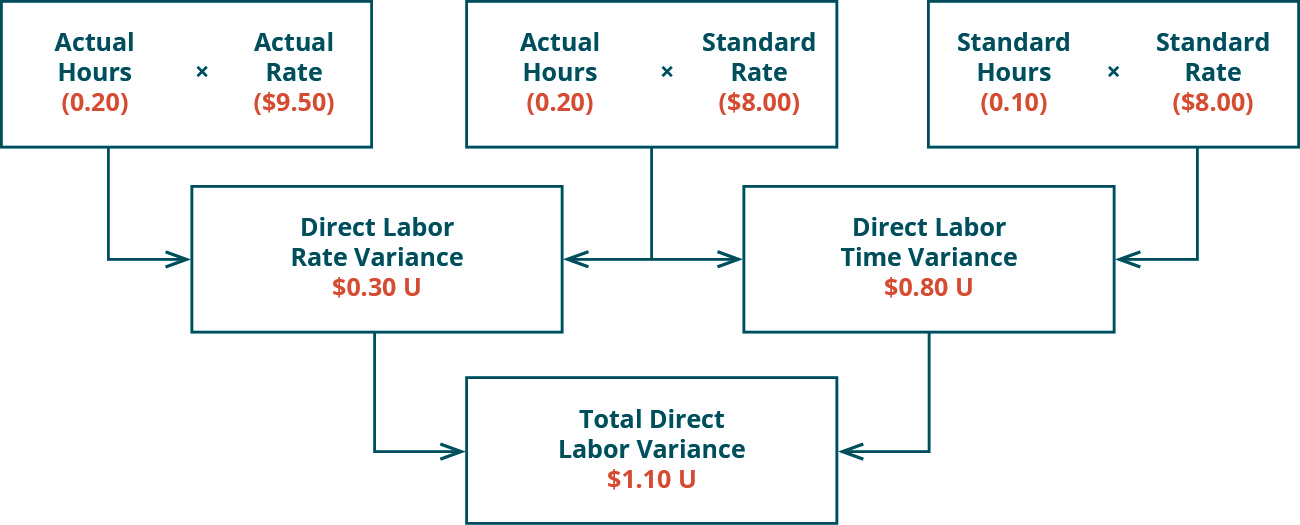As with the interpretations for the labor rate and time variances, the company would review the individual components contributing to the overall unfavorable outcome for the total direct labor variance, and possibly make changes to production elements as a result.

Sweet and Fresh Shampoo Labor

Biglow Company makes a hair shampoo called Sweet and Fresh. Each bottle has a standard labor cost of 1.5 hours at ?35.00 per hour. During May, Biglow manufactured 11,000 bottles. They used 16,000 hours at a cost of ?565,600. Calculate the labor rate variance, labor time variance, and total labor variance.

Solution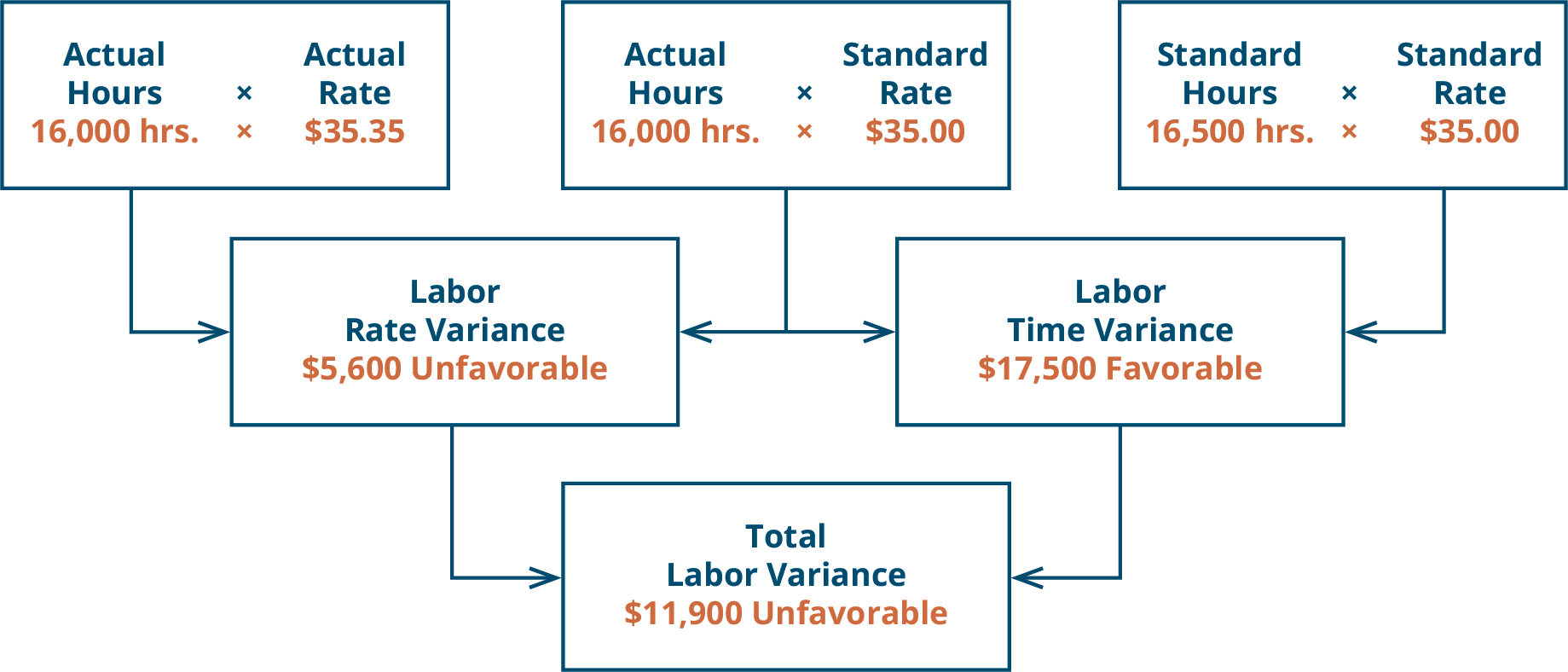Labor Costs in Service Industries

In the service industry, labor is the main cost. Doctors, for example, have a time allotment for a physical exam and base their fee on the expected time. Insurance companies pay doctors according to a set schedule, so they set the labor standard. They pay a set rate for a physical exam, no matter how long it takes. If the exam takes longer than expected, the doctor is not compensated for that extra time. This would produce an unfavorable labor variance for the doctor. Doctors know the standard and try to schedule accordingly so a variance does not exist. If anything, they try to produce a favorable variance by seeing more patients in a quicker time frame to maximize their compensation potential.

### Key Concepts and Summary

• There are two labor variances: the direct labor rate variance and the direct labor time variance.
• The direct labor rate variance determines if the rate paid is greater than or less than the standard rate.
• The direct labor time variance determines if the actual hours used are greater than or less than the standards that should have been used.

(Figure)What are some possible reasons for a labor rate variance?

1. hiring of less qualified workers
2. an excess of material usage
3. material price increase
4. utilities usage change

(Figure)When is the labor rate variance unfavorable?

1. when the actual quantity used is greater than the standard quantity
2. when the actual quantity used is less than the standard quantity
3. when the actual price paid is greater than the standard price
4. when the actual price is less than the standard price

C

(Figure)When is the labor rate variance favorable?

1. when the actual quantity used is greater than the standard quantity
2. when the actual quantity used is less than the standard quantity
3. when the actual price paid is greater than the standard price
4. when the actual price is less than the standard price

(Figure)What are some possible reasons for a direct labor time variance?

1. utility usage decrease
2. less qualified workers
3. office supplies spending
4. sales decline

B

(Figure)When is the direct labor time variance favorable?

1. when the actual quantity used is greater than the standard quantity
2. when the actual quantity used is less than the standard quantity
3. when the actual price paid is greater than the standard price
4. when the actual price is less than the standard price

(Figure)When is the direct labor time variance unfavorable?

1. when the actual quantity used is greater than the standard quantity
2. when the actual quantity used is less than the standard quantity
3. when the actual price paid is greater than the standard price
4. when the actual price is less than the standard price

A

(Figure)What is the direct labor rate variance?

A direct labor rate variance is the actual rate paid being different from the standard rate.

(Figure)What is the direct labor time variance?

(Figure)What are some possible causes of a direct labor rate variance?

Employees have a different level of experience than standards; the labor market is tighter or looser than expected; contract renegotiation.

(Figure)What are some possible causes of a direct labor time variance?

(Figure)How is the total direct labor variance calculated?

Total direct labor variance = (Actual hours × Actual rate) – (Standard hours × Standard rate) or the total direct labor variance is also found by combining the direct labor rate variance and the direct labor time variance.

(Figure)Queen Industries uses a standard costing system in the manufacturing of its single product. It requires 2 hours of labor to produce 1 unit of final product. In February, Queen Industries produced 12,000 units. The standard cost for labor allowed for the output was ?90,000, and there was an unfavorable direct labor time variance of ?5,520.

1. What was the standard cost per hour?
2. How many actual hours were worked?
3. If the workers were paid ?3.90 per hour, what was the direct labor rate variance?

(Figure)Penny Company manufactures only one product and uses a standard cost system. The following information is from Penny’s records for May: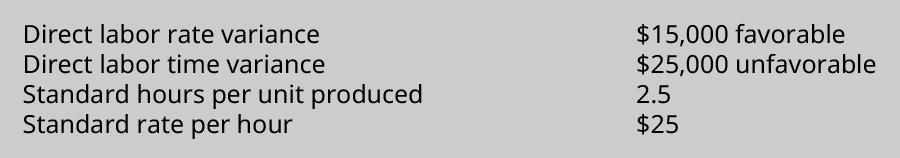During May, the company used 12.5% more hours than the standard allowed.

1. What were the total standard hours allowed for the units manufactured during the month?
2. What were the actual hours worked?
3. How many actual units were produced during May?

(Figure)Case made 24,500 units during June, using 32,000 direct labor hours. They expected to use 31,450 hours per the standard cost card. Their employees were paid ?15.75 per hour for the month of June. The standard cost card uses ?15.50 as the standard hourly rate.

1. Compute the direct labor rate and time variances for the month of June, and also calculate the total direct labor variance.
2. If the standard rate per hour was ?16.00, what would change?

(Figure)Eagle Inc. uses a standard cost system. During the most recent period, the company manufactured 115,000 units. The standard cost sheet indicates that the standard direct labor cost per unit is ?1.50. The performance report for the period includes an unfavorable direct labor rate variance of ?3,700 and a favorable direct labor time variance of ?10,275.
What was the total actual cost of direct labor incurred during the period?

(Figure)Ellis Company’s labor information for September is as follows: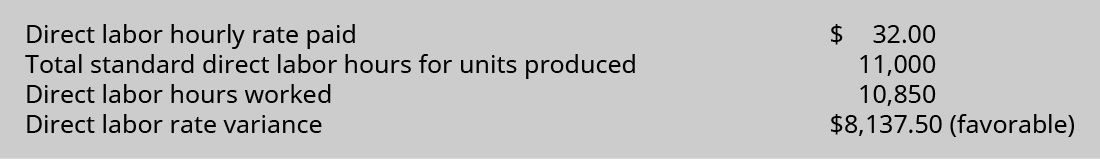1. Compute the standard direct labor rate per hour.
2. Compute the direct labor time variance.
3. Compute the standard direct labor rate if the direct labor rate variance was ?2,712.50 (unfavorable).

(Figure)Breakaway Company’s labor information for May is as follows: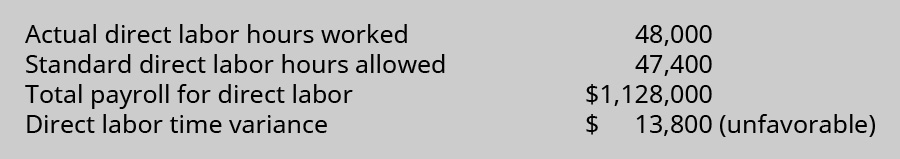1. What is the actual direct labor rate per hour?
2. What is the standard direct labor rate per hour?
3. What was the total standard direct labor cost for May?
4. What was the direct labor rate variance for May?

(Figure)Power Co.’s labor information for June is as follows: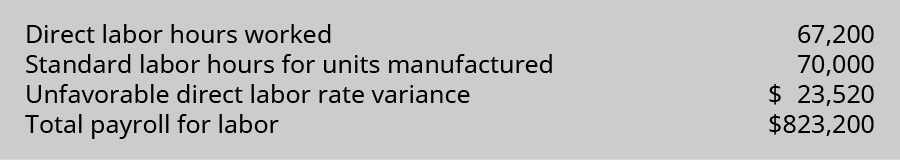1. What was the actual labor rate per hour?
2. What was the standard labor rate per hour?
3. What was the total standard labor cost for units produced in June?
4. What was the direct labor time variance for June?

(Figure)Marymount Company makes one product. In the month of April, it made 3,500 units. Workers were paid ?32 per hour for labor, for a total of ?718,848. The standard hours per unit are 6.4, and the standard labor wage rate is ?38.40 per hour.

1. What are the actual hours worked?
2. What are the standard hours for the units made?
3. What is the direct labor rate variance for April?
4. What is the direct labor time variance for April?
5. What is the total direct labor variance for April?

(Figure)Adam Inc.’s records for May include the following information: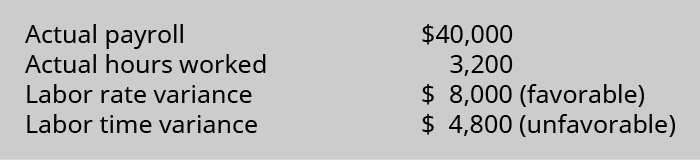2. What is Adam’s total standard labor cost for the units made?

(Figure)Ribco’s labor cost information for making its only product for March is as follows: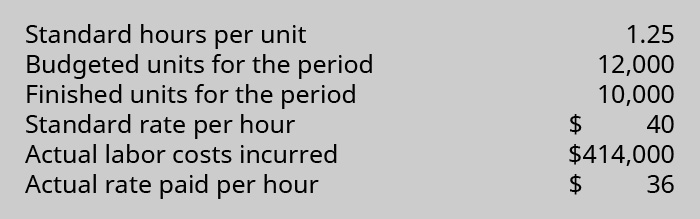1. What is the direct labor rate variance?
2. What is the direct labor time variance?
3. What is the total direct labor variance?

(Figure)Is labor a true variable cost?

### Footnotes

• 1Graham Kendall. “Why UPS Drivers Don’t Turn Left and Why You Shouldn’t Either.” The Conversation. January 20, 2017. http://theconversation.com/why-ups-drivers-dont-turn-left-and-you-probably-shouldnt-either-71432

### Glossary

direct labor rate variance
difference between the actual rate paid and the standard rate that should have been paid based on the actual hours worked
direct labor time variance
difference between the actual hours worked and the standard hours that should have been worked for the actual units produced
direct labor variance
measures how efficiently the company uses labor as well as how effective it is at pricing labor
total direct labor variance
actual labor costs compared to standard labor costs